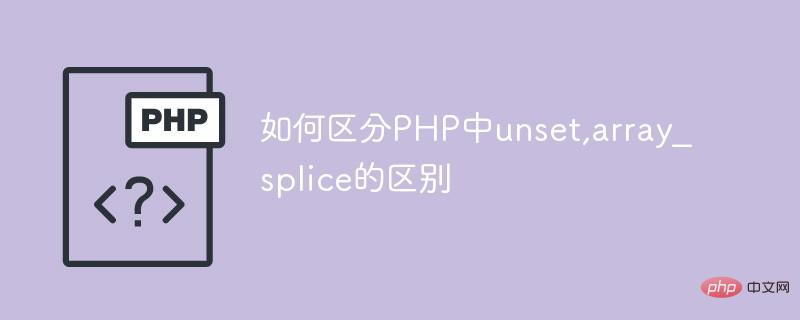# 如何区分PHP中unset,array_splice的区别逆旅行人2021-04-01 14:46:56原创1001.使用的函数

a.函数`unset（）`

`unset ( mixed \$var , mixed \$... = ? ) : void`

` unset()`销毁指定的变量。

b.函数`array_slice()`

`  array_splice(array,start,length,array)`
• array表示数组。

• start表示删除元素的开始位置。

• length表示被移除的元素个数，也是被返回数组的长度。(可选)

• array表示带有要插入原始数组中元素的数组（可选）

2.示例：

```<?php
\$arr = array('a','b','c','d');
unset(\$arr);
print_r(\$arr);
?>```

```Array
(
 => a
 => c
 => d
)```

```<?php
\$arr2 = array(1,3, 5,7,8);
foreach (\$arr2 as \$key=>\$value)
{
if (\$value === 3)
unset(\$arr2[\$key]);
}
var_dump(\$arr2);
?>```

```Array
(
 => a
 => c
 => d
)```### 全部评论我要评论

• 取消发布评论发送
• 1/1